Thermodynamics and Propulsion
Subsections

For a combustion process that takes place adiabatically with no shaft work, the temperature of the products is referred to as the adiabatic flame temperature. This is the maximum temperature that can be achieved for given reactants. Heat transfer, incomplete combustion, and dissociation all result in lower temperature. The maximum adiabatic flame temperature for a given fuel and oxidizer combination occurs with a stoichiometric mixture (correct proportions such that all fuel and all oxidizer are consumed). The amount of excess air can be tailored as part of the design to control the adiabatic flame temperature. The considerable distance between present temperatures in a gas turbine engine and the maximum adiabatic flame temperature at stoichiometric conditions is shown in Figure 3.24(b), based on a compressor exit temperature of(922 K).An initial view of the concept of adiabatic flame temperature is provided by examining two reacting gases, at a given pressure, and asking what the end temperature is. The process is shown schematically in Figure 15.3, where temperature is plotted versus the percentage completion of the reaction. The initial state is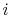and the final state is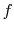, with the final state at a higher temperature than the initial state. The solid line in the figure shows a representation of the actual'' process.

To see how we would arrive at the final completion state the dashed lines break the state of reaction change into two parts. Process (1) is reaction at constantand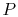. To carry out such a process, we would need to extract heat. Suppose the total amount of heat extracted per unit mass is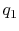. The relation between the enthalpy changes in Process (1) iswhereis the heat of reaction.''

For Process (2), we put this amount back into the products to raise their temperature to the final level. For this process,or, if we can approximate the specific heat as constant (using some appropriate average value)For the overall process there is no work done and no heat exchanged so that the difference in enthalpy between initial and final states is zero:The temperature change during this second process is therefore given by (approximately)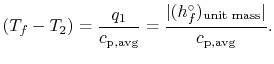(15..5)

The value of the adiabatic flame temperature given in Equation (15.5) is for 100% completion of the reaction. In reality, as the temperature increases, the tendency is for the degree of reaction to be less than 100%. For example, for the combustion of hydrogen and oxygen, at high temperatures the combustion product (water) dissociates back into the simpler elemental reactants. The degree of reaction is thus itself a function of temperature that needs to be computed. We used this idea in discussing the stoichiometric ramjet, when we said that the maximum temperature was independent of flight Mach number and hence of inlet stagnation temperature. It is also to be emphasized that the idea of a constant (average) specific heat,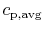, is for illustration and not inherently part of the definition of adiabatic flame temperature.

An example computation of adiabatic flame temperature is furnished by the combustion of liquid octane at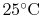with 400% theoretical air. The reaction is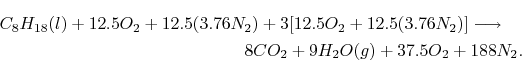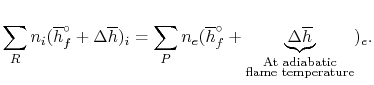(15..6)

We can again think of the general process in steps:
1. Bring reactants to[the term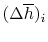] from the initial temperature, using whatever heat transfer,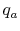, is needed. In this example we do not need step (i) because we are already at the reference temperature.
2. Reaction at[the term]. There will be some heat transfer in this step,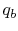, out of the combustor.
3. Put back heat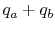into the products of combustion. The resulting temperature is the adiabatic flame temperature.

In the present case Equation (15.6) is, explicitly:We can examine the terms in the SFEE separately, starting with the heat of formation terms, and keeping track of units: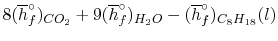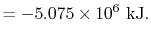The exit state at the adiabatic flame temperature is specified by: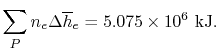We find the adiabatic flame temperature in three ways:
• an approximate solution using an average value of,
• a more accurate one using the tabulated evolution ofwith temperature,
• or a more accurate solution using the tabulated values for gas enthalpy in Table A.8 of SB&VW.

## 15.5.1 Approximate solution using average'' values of specific heat

From Figure 15.2 we can use the values at 500 K as representative. These are:

 Gas(kJ/kmole)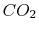4535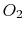30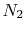30
Using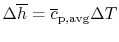,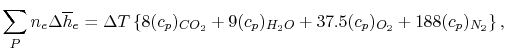where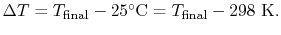and using the exit state calculated above, find that## 15.5.2 Solution for adiabatic flame temperature using evolutions of specific heats with temperature

Tables give the following evolutions of specific heats with temperature:

 Gas Evolution of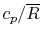with(kJ/kmol)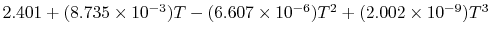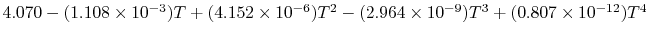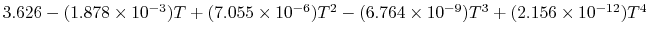Using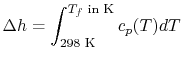and the same equation as above, we obtain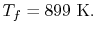## 15.5.3 Solution for adiabatic flame temperature using tabulated values for gas enthalpy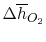28,041 21,924 19,246 18,221 kJ/kmole33,405 25,978 22,707 21,460 kJ/kmole

Plugging in the numbers shows the answer is between these two conditions. Linearly interpolating gives a value ofMuddy Points

Does adiabatic flame temperature'' assume 100% combustion? (MP 15.7)

What part of the computation for adiabatic flame temperature involves iteration? (MP 15.8)

UnifiedTP